DDC Programming Logic Symbols 1 - DDC programming in HVAC Control can be rather complex especially to those who don’t understand software or programming. Some software and hardware manufacturers utilize symbols to build their logic programs for HVAC DDC control. Some of these logic blocks are rather complex but others are very simple.

## DDC Programming Logic Symbols 1

These are the basic logic blocks that, when combined, make up the DDC algorithm control program. Everything from And gates to Or gates to Timers blocks to complex mathematical blocks allow a DDC Control programmer or DDC Control engineer to assemble programs in little time simple by arranging a set of logic blocks in a software program and then compiling this set of logic blocks into a basic machine line code and necessary tables which the casual observer and even the engineers never see. When this program is downloaded into the controller one can use the logic page in the DDC software to observe real-time information from inputs to outputs to alarms and schedules.

To someone skilled with this, they can use the “logic” page in the DDC software to troubleshoot problems. It is simply a tool along with trend charts and graphics to watch the system, collect data, and make changes as necessary or to use to find a problem in the program or in the HVAC or Electrical equipment which the program is controlling. We’ll start with simple blocks or symbols and work our way up to the more complex blocks. Understanding ladder diagrams and basic electrical circuitry is necessary to follow these logic gates and symbols.

### Binary Input – Analog Input – Binary Output – Analog Output

• The Binary input logic block will get an input signal of either on or off (0 or 1 in Binary speak) from a device wired to the DDC controller. It will send an appropriate response out through other logic blocks for program processing through other logic blocks and eventually end at an output block. An example of a Binary input logic block would be a Binary input logic block that gets its input from a smoke alarm. If the contacts on the smoke alarm close the Binary input logic block becomes true and sends this through the program for the appropriate response.
• The Analog input logic block with receiving an Analog signal from a device wired to the DDC controller. (see DDC Page 2 for an explanation of Analog and Binary signals). An example of an Analog input logic block would be a logic block that gets its input from a pressure transducer. The pressure transducer will send an analog signal to the Analog input logic block and the Analog input logic block will send this information through the program logic.
• The Binary output logic block will get its instructions from other logic blocks in the DDC program and give a binary output response based on the input from the program. An example of a Binary output logic block would be a Binary out logic block assigned to a motor start/stop. When the program called for the motor to energize the Binary output logic block would energize the output and really would energize the motor starter.
• The Analog output logic block will do the same as the Binary output logic block except the signal coming from the Analog output logic block will be an analog signal. An example of an Analog output logic block would be an Analog output logic block assigned to control a variable frequency drive which works off of an analog signal like 0 to 10 volts or 4 to 20 milliamps.

### And Gate | DDC Programming Logic Symbols 1The And gate is simply a series switch. In the DDC graphic to the left is an And gate and it is the same as the series switch to the right of the And gate. If a single input to the And gate is open the And gate will not allow an output. The input side of the And gate can have as many gates as necessary for proper functioning whatever is being controlled.

### Or Gate | DDC Programming Logic Symbols 1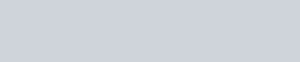The Or gate is simply a circuit that says “on” or the other (off) or both. If one or the other has an input signal to the Or gate the Or gate will allow an output. For example, you could have a three gate Or gate which would be linked to three specific alarms like the smoke detectors, the freeze stat, and the high static pressure switch. If one or the other tripped then the Or gate would send an output signal to an alarm logic block and an emergency shutdown logic block.

### The Not Gate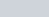The Not gate is a simple gate that will change a 0 input to a 1 output or a 1 input to a 0 output. It used for various logic programming to change a binary value from a 0 to a 1 or from a 1 to a 0

### If Less Than and If Greater Than Logic BlocksThis is basic line code programming that goes back to the basics of if, else, then. An example of the usage of these blocks would be if the outside air temperature was less than 65° then it is okay to use the economizer. Of course, the program would have other conditions which may be linked through an And Gate logic block but the If Less Than logic block would be one of the conditions to enable the economizer. There are other If logic blocks such as If Equals to and others that can give an analog or a binary output to the program.

### Low Limit Analog Logic Block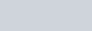The Low Limit analog signal block will place a low limit on an analog signal coming from the program going to the analog output. An example of this would be a minimum speed for a VFD or a minimum damper position for a modulating damper.

### High Limit Analog Logic BlockThe High Limit analog signal block will place a high limit on an analog signal coming from the program going to the analog output. An example of this would a high analog limit to the analog output of a damper that you only want to open to a maximum of 80%.

### Delay on Make Logic Block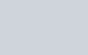Every control or HVAC control technician knows the value of a Delay on Make logic block. You can delay fans and compressors using this control logic block and prevent short cycling.

### Delay on Break Logic BlockAgain, every control or HVAC control technician knows the value of a Delay on Break circuit in HVAC Controls. You can delay a fan shut down or damper closing using this block.

### PID Logic BlockProportional (P), Integral (I), and Derivative (PID) control responses are very complex algorithms. This is the logic block that will help the DDC control program tune the device being controlled using PID tuning calculations and parameters. This logic block allows user input to tune different devices with PID control to prevent overshoot and undershoot. It prevents devices from under responding or over responding to program inputs and allows for smoother operation based on the PID control algorithm.

DDC Programming Logic Symbols

There are many more logic blocks to be covered. We will update the site with more logic blocks and an example of how some of these blocks would work together in a future update.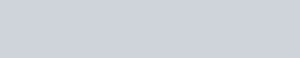DDC Programming Logic Symbols